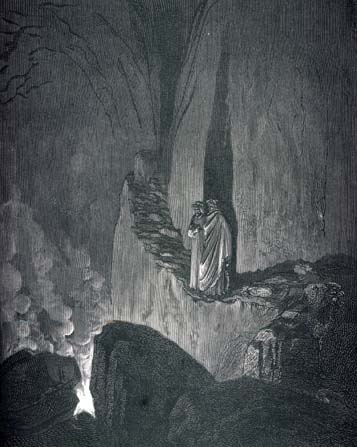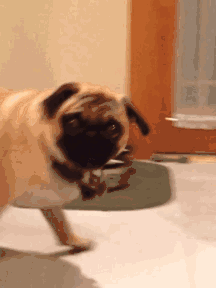# The Ghost Printer Behind Top-level R Expressions

### Yihui Xie 2017-06-08

For any developers who have ever written an S3 method for the `print()` function, they probably know what a top-level R expression means, but this is a very confusing concept to non-developers. I have to explain this every now and then, so I decided to write a short post about it.

Yesterday I saw a Github issue in the rmarkdown repository, and you can see that there are still users confused by the fact that ggplot2 plots are not rendered in certain cases. I have seen similar questions perhaps hundreds of times. Such questions have been answered in the R FAQ 7.22 “Why do lattice/trellis graphics not work?”, but the answer didn’t explain the root reason in detail.A top-level R expression is usually implicitly printed. Both words can cause confusion: printing is implicit so that you probably don’t consciously know it, and printing means the `print()` function is called on the object returned by the expression. For example, when you type `1 + 1` in the R console, and press Enter/Return, what actually happens is `print(2)`, where `2` is the value returned by `1 + 1`.

The reason that you create a `ggplot()` object in your R console and it shows up after you press Enter is that ggplot2 has defined a `print.ggplot` method on such objects. `ggplot()` does not actually create the plot – it only creates a `ggplot` object. It is the print method that actually creates the plot in a graphical device (as a side-effect).

Expressions nested in other expressions are not top-level expressions. For example, in a `for` loop, ggplot objects or HTML widgets or `knitr::kable()` cannot be displayed because they are not printed, unless you explicitly `print()` them.

Top-level R expressions are not always printed. They are not printed if they are marked as invisible. For example, if you run `invisible(1 + 1)` in the R console, you won’t see any value printed.

Many R functions return invisible values wrapped in the `invisible()` function. I want to show a few functions that you may have never recognized: `if`, `(`, and assignment operators such as `=` and `<-` are all functions. Let’s start from the assignment operator:

``````library(ggplot2)
p = ggplot(mpg, aes(cty, hwy)) + geom_point()
``````

This creates a ggplot object but you do not see the plot even though this is a top-level expression, and that is because the assignment operator `=` returns the value invisibly. If you type `p` in the console later, you will see the plot since `p` is implicitly printed.

You can force a top-level expression to be visible (hence printed) by wrapping it in `()`, e.g., `(invisible(1 + 1))` will be printed, and so will the ggplot object below:

``````library(ggplot2)
(p = ggplot(mpg, aes(cty, hwy)) + geom_point())
``````

The `if` function returns the value of a last expression depending on the condition. For example, `if (TRUE) A else B` returns `A`, and `if (FALSE) {A} else {B; C}` returns `C`. With that in mind, you will understand why the ggplot object is not displayed in the `if` statement below:

``````library(ggplot2)
if (TRUE) {
ggplot(mpg, aes(cty, hwy)) + geom_point()
1 + 1  # the value of this expression is returned (and printed)
}
``````

Note `for`, `while`, and `repeat` loops always return an invisible `NULL`, so nothing will be printed automatically, including the last expression in the loop, e.g.,

``````library(ggplot2)
for (i in 1:10) {
ggplot(mpg, aes(cty, hwy)) + geom_point()
}
``````

However, base R graphics is a different animal. All base R graphics functions will create plots no matter if they are called in top-level R expressions. For example, this will create 10 plots:

``````for (i in 1:10) {
plot(rnorm(100))
}
``````

WAT?WAT!Lastly, there is a function `withVisible()` that can tell you whether an expression returns a visible value or not, e.g.,

``````withVisible(1 + 1)
# \$value
#  2
#
# \$visible
#  TRUE

withVisible({x = 1 + 1})  # assignment in {}
# \$value
#  2
#
# \$visible
#  FALSE
``````

I hope you could remember the “ghost printer” the next time you run any R code in your R console or R Markdown or any knitr documents.